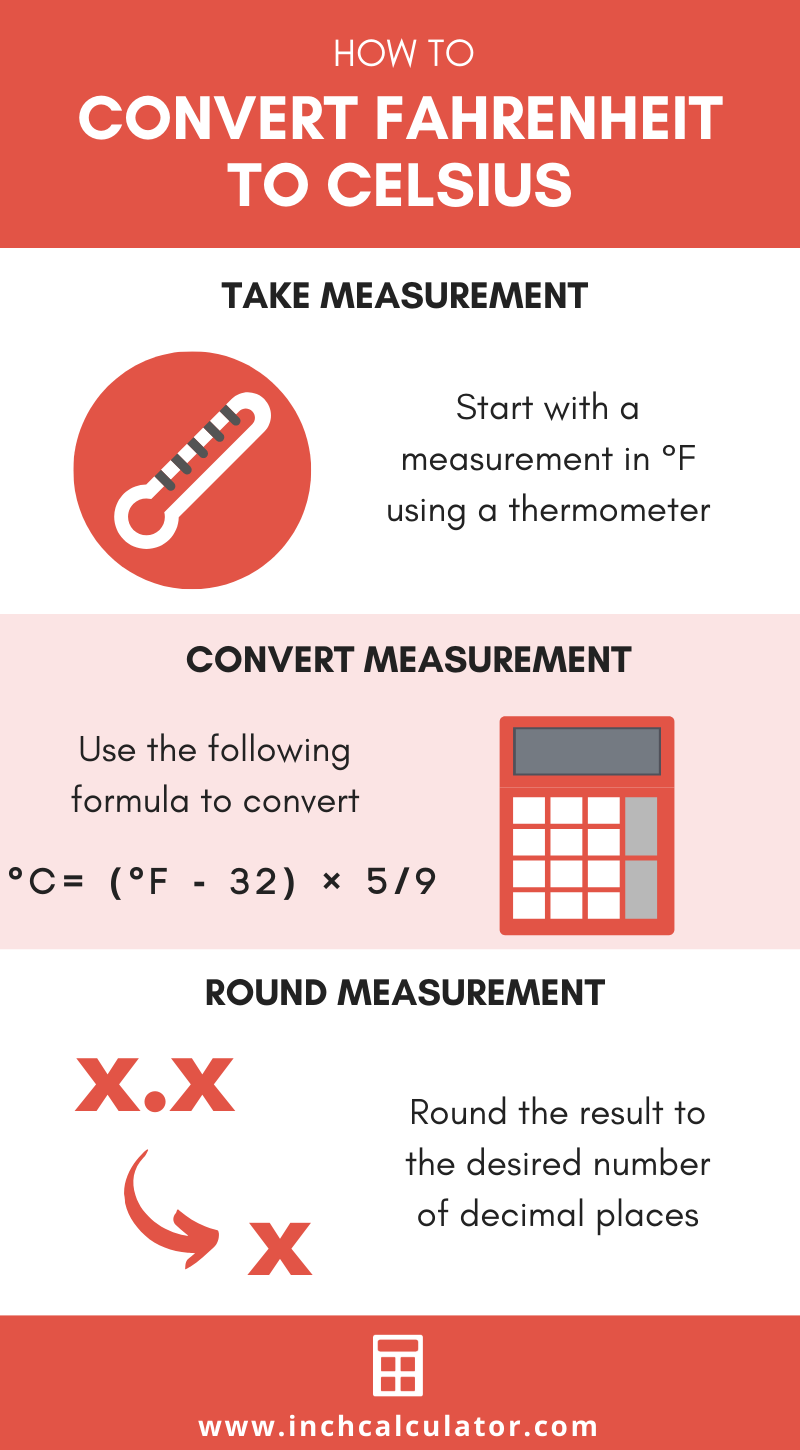# Fahrenheit to Celsius Converter (°F to °C)

Enter the temperature in Fahrenheit below to get the value converted to Celsius.

Results in Degrees Celsius:68 °F = 20 °C

Do you want to convert Celsius to Fahrenheit?

## How to Convert Fahrenheit to Celsius

There are a few formulas that you can use to convert degrees Fahrenheit to degrees Celsius.

### °F to °C Conversion Formula

Convert °F to °C using the widely accepted conversion formula:

°C = (°F - 32) × 5/9

The temperature in degrees Celsius is equal to the temperature in degrees Fahrenheit minus 32, times 5, divided by 9. Insert the °F temperature measurement in the formula and then solve to find the result.

For example, let's convert 50 °F to °C:

50 °F = (50 - 32) × 5/9
50 °F = 18 × 5/9
50 °F = 10 °C

### Alternate Fahrenheit to Celsius Formula

The National Institute of Standards and Technology actually defines the following formula for the conversion:

°C = (°F - 32) ÷ 1.8

Ultimately this is a reduced version of the widely accepted formula shown above.

## How to Convert a Temperature Without a Calculator

To convert Fahrenheit to Celsius without a calculator, you can use a simple rule of thumb. Subtract 30 from the temperature, then divide the result in half.

Note that this is not a precise method, but rather a quick shortcut to get a rough conversion when you don't have a calculator handy.

For example, let's use this shortcut to convert 70 °F to Celsius.

Start by subtracting 30 from the temperature.

70 °F - 30 = 40

Then, divide it in half.

40 ÷ 2 = 20

So, 70 °F is roughly equal to 20 °C using this method.Are you converting temperatures or cooking or baking? Try our oven temperature conversion calculator.

### Temperature Reference Points In °F and °C

Temperature reference points expressed in degrees Fahrenheit and Celsius
TemperatureDegrees FahrenheitDegrees Celsius
Absolute Zero-459.67 °F-273.15 °C
Freezing Point of Water32 °F0 °C
Triple Point of Water32.018 °F0.01 °C
Boiling Point of Water212 °F100 °C
Surface of the Sun10,100 °F5,600 °C

## What Is Fahrenheit?

The Fahrenheit scale is a temperature scale that defines the melting point of water as 32 degrees and the boiling point of water at 212 degrees. There are 180 intervals between 32 °F and 212 °F, each corresponding to one degree.

The degree Fahrenheit is a US customary and imperial unit of temperature. Fahrenheit can be abbreviated as F; for example, 1 degree Fahrenheit can be written as 1 °F.

## What Is Celsius?

The Celsius temperature scale, also commonly referred to as the centigrade scale, is defined in relation to the kelvin. Specifically, degrees Celsius is equal to kelvins minus 273.15.

The degree Celsius is the SI derived unit for temperature in the metric system. A degree Celsius is sometimes also referred to as a degree centigrade. Celsius can be abbreviated as C; for example, 1 degree Celsius can be written as 1 °C.

## Fahrenheit to Celsius Conversion Chart

Fahrenheit measurements converted to Celsius
Fahrenheit Celsius
-50 °F -45.56 °C
-40 °F -40 °C
-30 °F -34.44 °C
-20 °F -28.89 °C
-10 °F -23.33 °C
0 °F -17.78 °C
10 °F -12.22 °C
20 °F -6.6667 °C
30 °F -1.1111 °C
32 °F 0 °C
40 °F 4.4444 °C
50 °F 10 °C
60 °F 15.56 °C
70 °F 21.11 °C
80 °F 26.67 °C
90 °F 32.22 °C
100 °F 37.78 °C
110 °F 43.33 °C
120 °F 48.89 °C
130 °F 54.44 °C
140 °F 60 °C
150 °F 65.56 °C
160 °F 71.11 °C
170 °F 76.67 °C
180 °F 82.22 °C
190 °F 87.78 °C
200 °F 93.33 °C
300 °F 148.89 °C
400 °F 204.44 °C
500 °F 260 °C
600 °F 315.56 °C
700 °F 371.11 °C
800 °F 426.67 °C
900 °F 482.22 °C
1,000 °F 537.78 °C

## References

1. National Institute of Standards and Technology, SI Units – Temperature, https://www.nist.gov/pml/owm/si-units-temperature
2. National Oceanic and Atmospheric Administration National Weather Service , Fahrenheit, https://w1.weather.gov/glossary/index.php?word=fahrenheit
3. National Institute of Standards and Technology, SI Units - Temperature, https://www.nist.gov/pml/owm/si-units-temperature